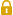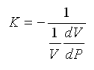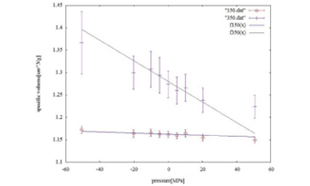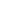Full Atomistic MD
Mechanical / Viscosity / Viscoelasticity
Materials ScienceUser Only

# Bulk Modulus Evaluation

Mechanical properties (volume elasticity modulus) can be evaluated using the MD engine COGNAC. Specifically, the volume changes of an existing system can be evaluated by calculating changes in pressure on the system. The resulting formula below is used to obtain the volume elasticity modulus.Generally, it is known that the volume elasticity modulus changes suddenly near the glass-transition temperature. We calculated the volume elasticity modulus of polyethylene (PE) in an amorphous state at a temperature near the glass-transition temperature (250 K). Figure 1 graphs the relationship between the PE pressure and volume obtained from the above calculations. The volume elasticity modulus values from this graph were estimated below for this calculation.

Temperature T = 150 [K] : Volume Elasticity Modulus K = 3.76841460651 [GPa]
Temperature T = 350 [K] : Volume Elasticity Modulus K = 0.664790332457 [GPa]Figure 1. Estimated PE Volume Elasticity ModulusThis website uses cookies to improve functionality and performance. If you continue browsing the site, you are giving implied consent to the use of cookies on this website. If you want to know more or refuse consent, read our Cookie Policy.

Accept